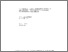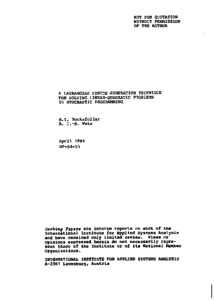# A Lagrangian Finite Generation Technique for Solving Linear-Quadratic Problems in Stochastic Programming

Rockafellar, R.T. & Wets, R.J.-B. (1984). A Lagrangian Finite Generation Technique for Solving Linear-Quadratic Problems in Stochastic Programming. IIASA Working Paper. IIASA, Laxenburg, Austria: WP-84-025Preview Text WP-84-025.pdf Download (1MB) | Preview

## Abstract

A new method is proposed for solving two-stage problems in linear and quadratic stochastic programming. Such problems are dualized, and the dual, although itself of high dimension, is approximated by a sequence of quadratic programming subproblems whose dimensionality can be kept low. These subproblems correspond to maximizing the dual objective over the convex hull of finitely many dual feasible solutions. An optimizing sequence is produced for the primal problem that converges at a linear rate in the strongly quadratic case. An outer algorithm of augmented Lagrangian type can be used to introduce strongly quadratic terms, if desired.

Item Type: Monograph (IIASA Working Paper) Adaption and Optimization (ADO) IIASA Import 15 Jan 2016 01:54 27 Aug 2021 17:12 https://pure.iiasa.ac.at/2493

### Actions (login required)View Item